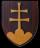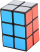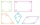# Inequation

Solve the inequation:

5k - (7k - 1)≤ 2/5 . (5-k)-2

k≥:  0.625

### Step-by-step explanation:Did you find an error or inaccuracy? Feel free to write us. Thank you!## Related math problems and questions:

• Equation with xSolve the following equation: 2x- (8x + 1) - (x + 2) / 5 = 9
• EquationSolve the equation: 1/2-2/8 = 1/10; Write the result as a decimal number.
• Equation with fractionsWhat is the simplified form of the equation fraction 4 over 5 n minus fraction 1 over 5 equals fraction 2 over 5 n? n = -2 n = 4 n = fraction 1 over 2
• Fractions and mixed numerals(a) Convert the following mixed numbers to improper fractions. i. 3 5/8 ii. 7 7/6 (b) Convert the following improper fraction to mixed number. i. 13/4 ii. 78/5 (c) Simplify these fractions to their lowest terms. i. 36/42 ii. 27/45 2. evaluate following ex
• Equation with fractionsSolve an equation: 1- (2x-5)/(6) =3- (x)/(4) It is an equation with fractions.
• If-then equationIf 1/2 + 2/5s = s - 3/4 what is the value of s?
• What is 9What is the value of x in the proportion 2 and one-fourth over x = 1 and one-half over 3 and three-fifths? 2 and two-fifths 5 and two-fifths 8 and 1 over 10 12 and 3 over 20
• Equation 29Solve next equation: 2 ( 2x + 3 ) = 8 ( 1 - x) -5 ( x -2 )
• Fraction of a NumberIf 1/2 of 1/3 of 1/4 of 1/5 of a number is 2.5. what is the number?
• Election mathematicsIn elections, 12 political parties received this shares of voters: party A 56.2 %party B 8.5 %party C 8.2 %party D 6.2 %party E 6.1 %party F 5.5 %party G 3.2 %party H 2.1 %party I 2 %party J 1 %party K 1 % Calculate what the shares acquired in the parliamA gift box has dimensions of: 8 1/2 inches, 5 1/2 inches, and 2 1/2 inches, respectively. How many cubes with side lengths of 1/2 inches would be needed to fill the gift box?Evaluate expression using BODMAS rule: 1 1/4+1 1/5÷3/5-5/8The number 135 split to two addends so that one addend was 30 greater than 2/5 the addend.Solve the following simple equation: 2. (4x + 3) = 2-5. (1-x)The perimeter of the quadrilateral a = 1m b = 14/5m c = 2 3/10m d = 1 4/5m?Solve following simple equation with fractions: -5/6(8+5b) = 75 + 5/3b1.5 divided by 1 = w divided by 4